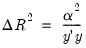User’s Guide : Basic Single Equation Analysis : Regression Variable Selection : Working with an Estimated Equation

Working with an Estimated Equation
Since variable selection is a pre-estimation tool, once estimated, the standard views and procs available for an equation estimated by least-squares will be available.
In addition, if Uni-directional or Stepwise was selected as the Selection Method, then a Selection Diagnostics item and several sub-menu items will be available on the equation View menu. These will allow you to examine how certain measures change during the selection process.
For both methods, there will be six sub-menu items: R-squared/t-Stats/Alpha-squared Graph, and R-squared/t-Stats/Alpha-squared Graph. The R-squared and t-Stats tables and graphs will show the selected value at each step. Alpha-squared is related to the change in R-squared at each step, namely:(22.2)
If Swapwise was selected as the Selection Method, then the Selection Diagnostics item will again be available, but only for R-squared and Alpha-squared.
If Auto-Search/GETS or Lasso was selected as the Selection Method, then a Model Selection Summary item and several sub-menu items will also be available on the equation View menu.
For both methods, there will be three sub-menu entries: Criteria Graph, Criteria Table, and Summary Path:
The Criteria Graph and Criteria Table items shows the model selection criteria for the twenty “best” candidate models. The graph form shows just the chosen criteria method (AIC, SIC or HQ). The table form of the view shows the log-likelihood value, the AIC, BIC and HQ values.
The Summary Path view provides a summary of the selection/dropping of variables along each selection path. For each path, the table provides the order in which variables were dropped, along with relevant selection information.
For Lasso, there are two additional sub-menu entries: Coefficient matrix, Lambda Coefficient Graph:
The Coefficient Matrix view is one of two views that allow you to easily see the behavior of the model over the entire lambda path. The leftmost column lists all the lambdas used in the estimation. The remaining columns display the coefficients at each value of lambda.
The Lambda Coefficient Graph displays values of coefficients ordered by the values of lambda in the lambda path. This is the graph you would see by plotting each column (excluding the constant, if included) of the View/Coefficient Matrix table against the leftmost lambda path column.